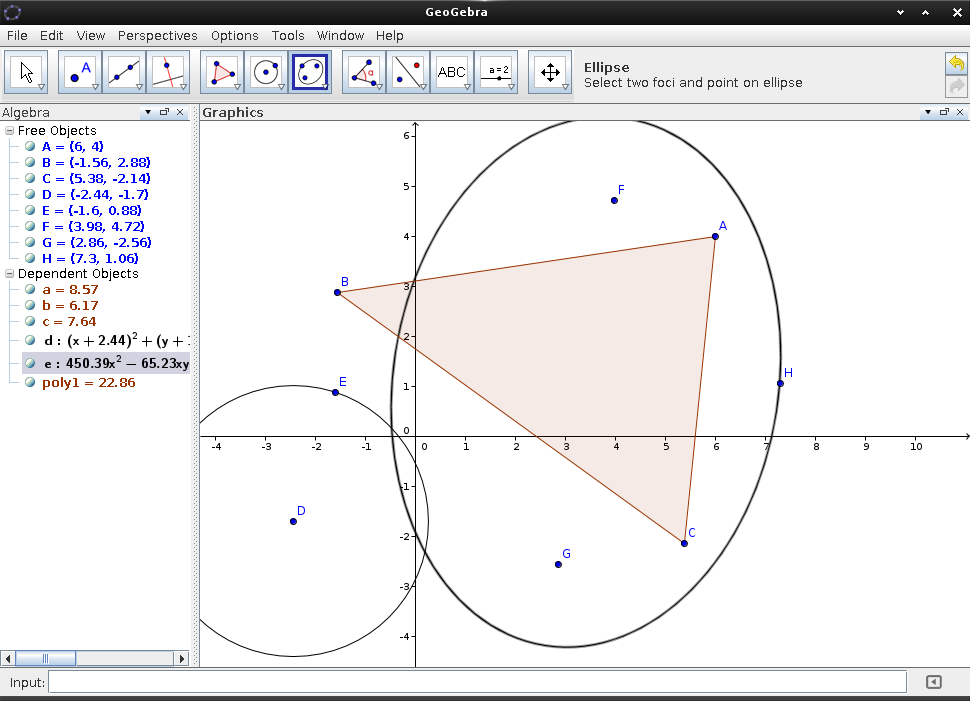Appcenter : Math and Science

## Featured: Geogebra## Equate

Equate is a simple calculator application written in C using the Enlightenment foundation libraries.

## Geogebra

GeoGebra is an interactive geometry, algebra, statistics and calculus application, intended for learning and teaching mathematics and science from primary school to university level.

## KAlgebra

KAlgebra is a mathematical graph calculator. While it is based on the MathML content markup language, knowledge of MathML is not required for use. The calculator includes numerical, logical, symbolic, and analytical functions, and...

## Qalculate!

Qalculate! is a multi-purpose desktop calculator for GNU/Linux. It is small and simple to use but with much power and versatility underneath. Features include customizable functions, units, arbitrary precision, plotting, and a user-friendly interface.

## Stellarium

Stellarium is a free open source planetarium for your computer. It shows a realistic sky in 3D, just like what you see with the naked eye, binoculars or a telescope. It is being used...

## Step

Step is an open source two-dimensional physics simulation engine. The software include the StepCore, a physical simulation library.

## wxMaxima

Maxima is a system for the manipulation of symbolic and numerical expressions, including differentiation, integration, Taylor series, Laplace transforms, ordinary differential equations, systems of linear equations, polynomials, sets, lists, vectors, matrices and tensors. Maxima...